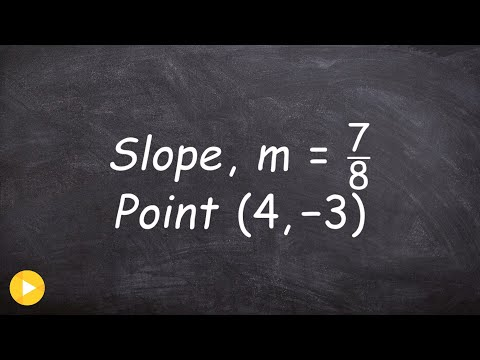# A line with a slope of -1/7 passes through the point (–7,7). What is its equation in slope-intercept form?

Relevance
• y - 7 = (-1/7)(x - - 7)

y - 7 = -x/7 - 1

y = -x/7 + 6

or

y = (-1/7)x + 6

•Login to reply the answers
• Point: (-7, 7)

Slope: -1/7

Equation: y -7 = -1/7(x - -7) => y = -1/7x +6

•Login to reply the answers
• Anonymous
1 month ago

Just follow the method shown here:•Login to reply the answers